This is in continuation to our last blog post, Trigonometry – Understanding Ratios, in which we discussed about trigonometric ratios (or functions). If you haven’t read that already, please have a look. It will set the baseline for this blog’s discussion.

Let’s move further and talk about properties of Trigonometric functions.

Periodic property:

What is periodic? Anything which repeats after a regular interval of time is periodic. That pendulum clock in your house – periodic. That swing in your nearby park – periodic.

Likewise, all 6 trigonometric functions are also periodic. The time after which it repeats itself is called “period” (or time period) of that function. Graph between one such period is called cycle.

For Sin, Cos, Csc and Sec – period isor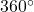While for Tan and Cot – period is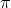orFrom this information, we come to another identity:where k is an integer and represents the number of cycles. (Same follows for Cos, Csc and Sec)where k is an integer and represents the number of cycles. (Same follows for Cot)

E.g:

Find the value of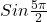I have covered some examples in the video below.

Odd-Even property:

This is a pretty straight forward property.

If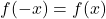, then f(x) is an even function

If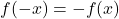, then f(x) is an odd function

Even functions are symmetric about y-axis and odd function are symmetric about origin.

Sin, Cos, Tan and Cot are odd functions while Only Cos and Sec are even functions.

I have covered some examples in the video below.

Sign of Trigonometric functions in different quadrants:

Coordinate plane is divided in 4 quadrants, we know this very well. Sign of each trigonometric function is defined in each quadrant.

In Quadrant 1 – All 6 trigonometric functions are positive

In Quadrant 2 – Only Sin and Csc are positive

In Quadrant 3 – Only Tan and Cot are positive

In Quadrant 4 – Only Cos and Sec are positive

E.g.: What is the sign of?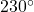is in Quadrant 3 (More thanbut less than), so< 0.

Other identities:

These identities can be used to convert one trigonometric function to other.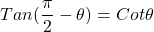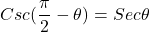Corollary of periodic property:.

Likewise,whereis any angle(acute/obtuse/right) and n is an integer. But how to decide about + or – ? You will find formulas in books but there is another conceptual way to learn this without the use of formulas. Check it out in this video:

Stay tuned for further blog posts.

Having questions? Post it down in the comment section.